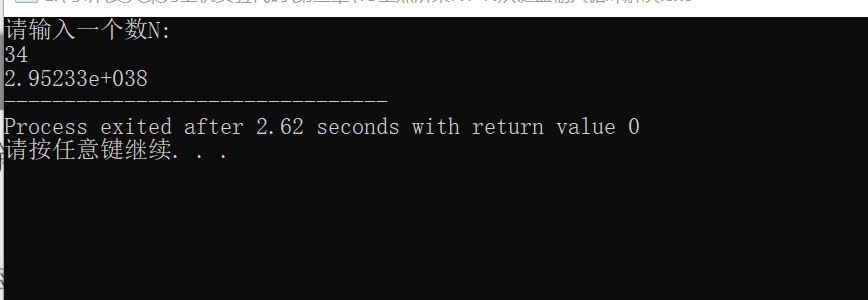• 求n阶乘（用三种不同的循环实现，提示：先从键盘输入n的值）while do while和forc# c语言
• N从键盘输入循环解决 #include<iostream> using namespace std; int main() { int n;//输入的数n double x=1; //累计的值,要用double，用int范围太小，算的少 cout<<"请输入一个数N...
西安交通大学梁力上机实验第三章15zd
15阶乘N！N从键盘输入，循环解决


#include<iostream>

using namespace std;

int main()
{

int n;//输入的数n

double  x=1;
//累计的值,要用double，用int范围太小，算的少

cout<<"请输入一个数N:" <<endl;

cin>>n;
/*递归用循环一定要注意
int i是从1开始，<= n，不然结果会是0
*/
for(int i=1;i<=n;i++)
{
x=x*i;
//累乘的结果是x=x*i,x要不断的使用，
//这次的结果，要用到下一次，=的左右都有x

}

cout<<x;

return 0;
}


结果如果对你有帮助，请 点赞 支持一下，谢谢！！！
展开全文• 当你的能力匹配不了你的野心时候,是该静下心来提深自己!...小编以10的阶乘为例，给大家分享下阶乘法： #include &lt;stdio.h&gt; int prime(int x); void main(){ int m,n; prin...
阶乘的定义：所有小于及等于该数的正整数的积
典型的递归算法，f(n)=n*f(n-1)
我们要C语言可以快速的进行编程计算，那么阶乘怎么求呢？小编以求10的阶乘为例，给大家分享下阶乘的求法：
#include <stdio.h>
int prime(int x);
void main(){
int m,n;
scanf("%d",&m);
n=prime(m);
printf("%d! 的阶乘是: %d\n", m,n);
}
int prime(int x){
int s;
if(x>0){
s=prime(x-1)*x;
}
else{
return 1;
}
return s;
}
作者声明：本篇经验系本人依照真实经历原创，未经许可，谢绝转载。https://blog.csdn.net/qq_42680327
展开全文递归
• 首先引入Scanner工具包，n阶乘是 1234…*n 具体看代码： import java.util.Scanner; public class NtLx1{ ... //控制台输入一个数字n, n阶乘 Scanner sc = new Scanner(System.in); System.out.pri...
首先引入Scanner工具包，n的阶乘是 1*2*3*4*....*n 具体看代码：
import java.util.Scanner;
public class NtLx1{
public static void main(String[] args){
//控制台输入一个数字n,求 n的阶乘
Scanner sc = new Scanner(System.in);
System.out.print("请输入你要计算阶乘的数：");
int n = sc.nextInt();
int sum = 1;
for(int i = 1;i<= n;i++){
sum *= i;
}
System.out.println(n+"的阶乘是："+sum);
}
}

展开全文• 问题描述 “从键盘输入n,1+2!+3!+...+n!的和”对于此题，我们可以用定义一个函数来解决，接着用一个for循环语句来设置1到n，接下来一起来编写这个代码吧。 解决方案 假定这个函数名称为f def f(x): f = 1 for i...广告关闭
腾讯云11.11云上盛惠 ，精选热门产品助力上云，云服务器首年88元起，买的越多返的越多，最高返5000元！
代码：def fei(i,j): #i为行数，j为列数 if i == 1 or j ==1:return 0 elif j == 2 : return 1 else:return fei(i,j-1) + fei(i,j-2) for i in range(1,7):print() for k in range(1,7-i):#控制空格数 print( ,end=) for j in range(1,(2*i)): print(fei(i,j),end=)运行结果：? 以上这篇python递归函数求n的阶乘...
i = int(input(input))sum = 0if i...
如h阶乘，就表示为h!； 阶乘一般很难计算，因为积都很大。 提问：求1+2! +3!+...+20! 的和实现环境：python3编辑器:pycharm分析：1、阶乘的计算就是比较麻烦的一部分，用递归函数实现是比较好的方案，先定义一个递归函数实现求阶乘功能。 defrecursion(n): 定义递归函数实现求阶乘功能ifn==1:return1else:returnn*...
问题本身很简单，主要是通过这个小问题来演示python的一些用法，例如测试代码运行时间、函数嵌套定义等等。 from time import timefrom math import factorialfrom functools importreducefrom random import randintdef myfactorial1(n):使用传统方法计算阶乘 result = 1 for i in range(1, n+1):result = result*i ...
文中给出了2段代码，在实际使用时应优先考虑使用第一段，第二段仅用来验证，涉及大量重复计算，效率极低。 def factorialbefore(n): result, t = 1, 1 for i in range(2, n+1):t *= i result += t return resultdef verify(n):from math import factorial result = 0 for i in range(1, n+1):result += factorial(i) ...main()求阶乘一个正整数的阶乘（factorial）是所有小于及等于该数的正整数的积，并且0的阶乘为1源码(python实现):def factorial(num): if num == 1 or num...在三根柱子之间一次只能移动一个圆盘源码(python实现):def hanoi(n, a, buffer, c):if(n == 1):print(a,--->,c) return hanoi(n-1, a, c, buffer) hanoi(1...问题描述 “从键盘输入n,求1+2!+3!+...+n!的和”对于此题，我们可以用定义一个函数来解决，接着用一个for循环语句来设置从1到n，接下来一起来编写这个代码吧。 解决方案 假定这个函数名称为f def f(x): f = 1 for i in range(1,x+1):f *= i return f n = int(input(“请输入正整数：”))print(“和为：%d“ % sum(map...
usrbinenv python# _*_ coding: utf-8 def factorial(n): if n == 0: return 1 else:return n * factorial(n - 1) print factorial(5)结果120总结：求阶乘问题算是递归中最简单的问题了。 源码地址：求阶乘，记得给个star。 参考资料程序设计与算法（二）算法基础...
用python3编程，求1! +2!+3!+...+n! 的和#用python3编程，求1! +2!+3!+...+n! 的和。 sum=0fac=1n=int(input(number=?))for i in range(1,n+1): fac*=i sum+=facprint(sum)...
递归——每调用一个函数，都会创建一个新的命名空间，意味着当函数调用自身时，实际上调用的是两个不同的函数 阶乘python代码1. >>>deffactorial(n):2. ifn==1:3. return14. else:5. returnn*factorial(n-1)6. 7. >>>factorial(5)8. 120 幂python代码1. >>>defpower(x,n):2. ifn==0:3. return14. else:5. returnx*...
知道公式后就很简单了，利用for循环，第几行i+1就等于几，当然python中是没有直接运算组合数的，这就需要自己分步计算。 只需要写两个函数，一个是排列运算函数，一个是阶乘函数，具体代码如下。 代码示例：def jiang(x): if x == 0: return 1 else:return x*jiang(x-1)def jiang1(n,x): sum = 1 for i in range(x)...
return 找不到这个值 ret= find(l,44)print(ret)# 参数 end# 返回值#找不到的话怎么办 # l.index() # 67 发生两次调用# 66 发生好几次# 44 找不到 #age，二分查找，三级菜单的代码看一遍# 斐波那契 # 问第n个斐波那契数是多少# 阶乘 #3！ 3*2*1# 附加题 ：考试附加题 # 递归实现# l = # print(l) # 超过最大递归限制...
当参数为不可变参数时，函数不会影响外部实参值，当参数为不可变参数时，函数则会影响外部实参值。 函数本身可作为参数传递。 python 中使用lambda 来实现匿名函数。 练习1、求n的阶乘def factorial(num): 求阶乘 :param num: 非负整数 :return:num的阶乘 result = 1 for n in range(1, num + 1):result *= n return ...import numpy as np>>> np.__version__1. 17. 0>>> np.hypot(3,4)5.0>>> def factorial(n): # 计算n的阶乘 if n == 0:# 递归出口 return 1 return n*...我始终认为，学习是需要沉下心来慢慢钻研的，是长期的； 同时，学习不应该被赋予太多的功利色彩。 一个python 程序员的成长路线图应该是这样子的...
下面是一个简单的例子：test= print(dir(test))12. 简化 if 语句 我们可以使用下面的方式来验证多个值：if m in :而不是：if m==1 or m==3 or m==5 or m==7:或者，对于 in 操作符我们也可以使用 ‘{1,3,5,7}’ 而不是‘’，因为 set 中取元素是 o(1) 操作。 13. 一行代码计算任何数的阶乘python 2.x.result=...
x - y} print(stdcalc(9,3))print(stdcalc(9,3)) #1-> 12#2->6一行代码计算任何数字的阶乘# python 2.x result =(lambda k: reduce(int.__mul__, range(1,k+1),1))(3)print(result)#-> 6 # python 3.x import functoolsresult =(lambda k: functools.reduce(int.__mul__, range(1,k+1),1))(3)print(result) #-> 6找到...
大于1的数n的阶乘是n乘n-1的阶乘。 def factorial(n): if n == 1: return 1 else:return n * factorial(n-1)=====计算幂例子：power(x,n)(x为n的幂次）是x...python中有两类参数：位置参数 和 关键数参数。 参数在给定默认值时是可选的。 作用域。 变量存储在作用域（也叫作命名空间）中。 python有两类主要的作用...
由于栈的大小不是无限的，所以，递归调用的次数过多，会导致栈溢出#递归实现阶乘n! = (n-1)! × ndef factorial(n): if n == 1: return 1 else:return n *...info(derek,22,cn,python) #derek 22 (cn, python)5. **kwargsdef info(name, *args, **kwargs):# **kwargs 会把多传入的参数变成一个dict形式 print(name...
python递归函数谢尔宾斯基三角形python第二十二课：python递归函数常见的递归拍照python第二十二课：python递归函数python递归代码实例递归求阶乘所谓的求...(n,fac(n)))注：在python3中，递归的深度被限制为100，如需改变其深度，需要import syssys.setrecursionlimit(10000) #10000为递归的深度...要做到这一点，你可以使用下面的代码片段。 它还以可读格式打印当前使用的python版本。 打印（“对不起，你没有在python 3.5上运行 n”打印（“对不起，你没有在python 3.5上运行 n”）打印（“请升级到3.5。 n”）打印（“请升级到3.5。 n” ）sys.exit（1）。 退出（1 ）＃以可读格式打印python版本。 ＃以可读格式...
展开全文• 从键盘输入一个数，出这个数的阶乘，即 n!。算法思想首先要清楚阶乘定义，所谓 n阶乘，就是 1 开始乘以比前一个数大 1 的数，一直乘到 n，用公式表示就是：1×2×3×4×…×(n-2)×(n-1)×n=n!具体的操作:...
• 本题初看没有什么问题，但是如果用int编程的话，输入20的时候数据会溢出，所以我们这里采用long long 的类型来编写。 #include<iostream> using namespace std; int main() { long long n,s=1; cout<&...
• 从键盘输入一个整数n,n项的阶乘之和，1+2!+3!+…+n!的和 输人样例 20 输出样例 2561327494111820313 代码： 首先了解什么是阶乘阶乘1乘以2乘以3乘以4一直乘到所要求的数。 通俗的讲也就是按顺序一...python 程序设计
• 从键盘输入一个数，出这个数的阶乘，即 n!。 算法思想 首先要清楚阶乘定义，所谓 n阶乘，就是 1 开始乘以比前一个数大 1 的数，一直乘到 n，用公式表示就是：1×2×3×4×…×(n-2)×(n-1)×n=n! 具体的操作:...c语言 算法 机器学习 数据结构
• 从键盘输入一个数，出这个数的阶乘，即 n!。 算法思想 首先要清楚阶乘定义，所谓 n阶乘，就是 1 开始乘以比前一个数大 1 的数，一直乘到 n，用公式表示就是：1×2×3×4×…×(n-2)×(n-1)×n=n! 具体的...编程 实例
• ## C语言求n的阶乘（n!）

千次阅读 多人点赞 2019-10-07 06:56:50
从键盘输入一个数，出这个数的阶乘，即 n!。 算法思想 首先要清楚阶乘定义，所谓 n阶乘，就是 1 开始乘以比前一个数大 1 的数，一直乘到 n，用公式表示就是：1×2×3×4×…×(n-2)×(n-1)×n=n!具体的...
• 1.求n阶乘 n阶乘=n*n-1*n-2*n-3...*2*1 注意要考虑输入的为0 或者1 的情况。 主要代码： #define _CRT_SECURE_NO_WARNINGS //为解决scanf函数不安全的警告问题 #include&lt;stdio.h&gt; #include&...
• package hello;import java.util.Scanner;public class Hello {public static void main(String[] args) { Scanner in = new Scanner(System.in); int n; n=in.nextInt(); int i=1,sum=1;... while(i<=n)java
• Python实现判断多次输入的年份是否是闰年 要想实现这个程序,先要理解什么是阶乘。...先定义一个变量n,因为阶乘是1乘2乘3…一直乘到n,所以这里用循环会好理解一点,再定义一个sum=1,将每循环一次得到的乘积赋值给s...python 编程语言 算法
• #include <stdio.h> #include <stdlib.h>... int i,product = 1 ,n; printf("please enter number "); scanf("%d",&n); for(i = 1 ; i <= n ; i++) { product *= i; }...
• 要求说明：3！= 3*2*1,5！=5*4*3*2*1，依次类推 n！...//用while语句求阶乘n！#include &lt;stdio.h&gt;int main(int argc, char **argv){ int n ; int i = 1; printf("Input n:\n"); scan...
• //递归实现n阶乘 import java.util.Scanner; public class Factorial1{ //实现阶乘的方法——使用递归 //要接收一个参数，应该定义参数类型和名字 int i //需要n阶乘，是一个数字，应该有返回值，返回值类型int...
• 首先求n阶乘，我们先要产生1-n的数字，然后累乘起来，利用for循环来实现。 所以求n阶乘的具体代码如下：#include &lt;stdio.h&gt; #include &lt;stdlib.h&gt; int main() { int i = 0; int n...
• 从键盘输入任意一个大于等于0的整数n，然后计算n阶乘，并把它输出。 提示： 0！是 1 。 Input 输入任意一个大于等于0的整数n。 Output 输出n！ Sample Input 3 Sample Output 6 #include <stdio.h> int main...
• 从键盘输入一个正整数 n, n! #include <stdio.h> int main () { int i = 2 , n; float fac = 1; printf("请输入一个正整数：\n"); scanf("%d", &n); if(n == 0 || n == 1) { printf("%d 的阶乘...
• Ⅰ、用do-while求n阶乘： ①、//代码摘下直接可以运行 //n阶乘(n!) #include<stdio.h> int main() { int t,n,result; result=1; printf(“Please input the number of n!\n”); scanf("%d",&n); t=n; ...编程语言 c语言
• 计算阶乘 编写程序，输入整数 n，计算并输出 n阶乘n!=1×2×3×⋯×n 其中：0≤n≤20 本程序修改result数组大小可计算n>20的阶乘
• for循环 #include <... printf("请输入数字：\n"); scanf_s("%d", &n); for (i = 1; i <= n; i++) { sum *= i; printf("%d的阶乘是%d\n", i,sum); } return 0; } while循环c语言
• //输入一个整数，阶乘 //作者：wsg int main(void) { int a; printf("Please input integer a:\n"); scanf("%d", &a); int i = 1; int num = 1; //使用for循环 /************* for( ; i; i++) { ...C语言
• 输入一个整数n出其阶乘 #include <iostream> #include <cstdio> using namespace std; int main() { int a=1,n; printf("输入整数n的值:"); scanf("%d",&n); while(n>0) { a=n*a; n=n-...c语言 算法
• 输入一个整数n它的阶乘。 注：0！= 1 源代码： 方法一：递归 #include <stdio.h> #include <stdlib.h> int fack(int n) { if(n == 0) { return 1; } return n*fack(n-1); } int main() {...C语言
• 从键盘输入任意一个大于等于0的整数n，然后计算n阶乘，并把它输出。 提示： 0！是 1 。 Input 输入任意一个大于等于0的整数n。 Output 输出n！ Sample Input 3 Output 6 import java.util.Scanner; public class ......